# CBT JAMB MATHS 2017

/47
84
Created on By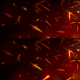bola

Maths JAMB CBT 2017

1 / 47

1. Given T = { even numbers from 1 to 12 }

N = {common factors of 6, 8 and 12}

Find T ∩ N

2 / 47

2. What is the next number in the series 2, 1, 1/2, 1/4

3 / 47

3. If U = {x : x is an integer and 1 ≤ x ≤ }

E1 = {x: x is a multiple of 3}

E2 = {x: x is a multiple of 4} and an integer is picked at random from U, find the probability that it is not in E2

4 / 47

4. The curved surface area of a cylinder 5cm high is 110cm2. Find the radius of its base. π = 22/7

5 / 47

5. If two graphs Y = px2 + q and y = 2×2 − 1 intersect at x =2, find the value of p in terms of q

6 / 47

6. Evaluate (sin45 + sin3 ) in surd form7 / 47

7. If y = x Sin x, find  dy/dx  when x = π/2

8 / 47

8. If temperature t is directly proportional to heat h, and when t = 20oC, h = 50 J, find t when h = 60J

9 / 47

9. Evaluate 1 – ( 1/5 x  2/3) + ( 5 + 2/3)

10 / 47

10. Given m = N (√SL/T ) make T the subject of the
formula

11 / 47

11.  Simplify 3n-1 × 27n+1/81n

12 / 47

12. The locus of a point which is equidistant from
the line PQ forms a

13 / 47

13. Given T = {even numbers from 1 to 12}
N = {common factors of 6, 8 and 12} Find T, N

14 / 47

14. Given the quadrilateral RSTO inscribed in the circle
with O as centre. Find the size angle x and given
RST = 60 degrees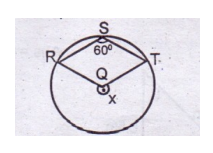15 / 47

15. Find the sum of the range and the mode of the
set of numbers 10, 9, 10, 9, 8, 7, 7, 10, 8, 10, 8, 4, 6,
9, 10, 9, 7, 10, 6, 5

16 / 47

16. Find the sum to infinity of the series 1/4, 1/8, 1/16,……….

17 / 47

17. The base in which the operation was performed was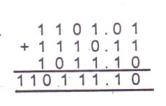18 / 47

18. The value of x + x ( x x ) when x = 2 is

19 / 47

19. In a regular polygon, each interior angle doubles
its corresponding exterior angle. Find the number of
sides of the polygon

20 / 47

20. A cylindrical tank has a capacity of 3080m 3 .
What is the depth of the tank if the diameter of its
base is 14m? Take pi = 22/7.

21 / 47

21. Simplify 4 √27 + 5 √12 − 3√75

22 / 47

22. A man covered a distance of 50 miles on his first
trip, on a later trip he traveled 300 miles while going
3 times as fast. His new time compared with the old
distance was?

23 / 47

23. In the figure, find x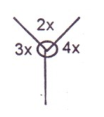24 / 47

24. Divide 4x 3 – 3x + 1 by 2x – 1

25 / 47

25. A car dealer bought a second-hand car for of
250,000 and spent N 70,000 refurbishing it. He then
sold the car for N400,000. What is the percentage
gain?

26 / 47

26. Find the number of ways that the letters of the
word EXCELLENCE be arranged

27 / 47

27. Evaluate (0.00000231/0.07) and leave the answer in
standard form

28 / 47

28. If a rod 10cm in length was measured as 10.5cm,
calculate the percentage error

29 / 47

29. Find the principal which amounts to ₦ 5,500 at a
simple interest in 5 years at 2% per annum

30 / 47

30. The pie chart shows the allocation of money to each
sector in a farm. The total amount allocated to the
farm is ₦ 80 000. Find the amount allocated to
fertilizer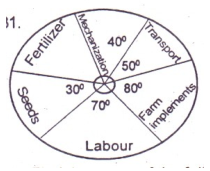31 / 47

31. In how many ways can the word
MATHEMATICS be arranged?

32 / 47

32. In how many ways can the word MACICITA be
arranged?

33 / 47

33. y is inversely proportional to x and y and 6 when
x = 7. Find the constant of the variation

34 / 47

34. In the diagram MN, PQ and RS are parallel lines.
What is the value of the angle marked X?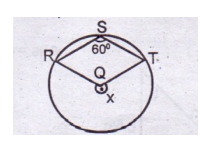35 / 47

35. Find the equation of the locus of a point p (x, y)
such that pv = pw, where v= (1, 1) and w = (3, 5)

36 / 47

36. Find ∫(x 2 + 3x − 5)dx

37 / 47

37. If m * n = [m n − n m ] for m, n belong to R,
evaluate − 3 * 4

38 / 47

38. Factorize completely x 2 + 12xy + y + 3x + 3y -18

39 / 47

39. Make S the subject of the relation, p = s + (sm2 /nr)

40 / 47

40. The operation * on the set R of real number is
defined by x * y = 3x + 2y − 1, find 3* − 1

41 / 47

41. Find the gradient of the line joining the points (3,
2) and (1, 4)

42 / 47

42. Simplify (3√64a 3 )−1

43 / 47

44. If  (2√3 – √2 / √3 + 2√2) = m + n√6,

find the values of m and n respectively

44 / 47

44. If α and β are the roots of the equation 3x 2 + bx – 2 = 0.

Find the value of 1/α + 1/β

45 / 47

45. Find the range of the following set of numbers
0.4, −0.4, 0.3, 0.47, −0.53, 0.2 and −0.2

46 / 47

46. Evaluate 1 − ( 1/5 + 12/3 ) + (5 + 12/3 )

47 / 47

47. What is the product of 2x 2 − x + 1 and 3 − 2x